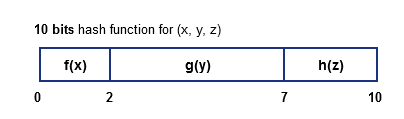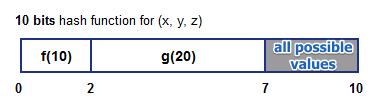# ML Wiki

## Partitioned Hash Function Index

### Typical Approach

Suppose we use classical approach for hashing a tuple $(a, b)$

• $h(a, b) = h_1(a) + h_2(b)$
• in this case we need to provide both $a$ and $b$
• but what if we want to query only for $a$?
• this will not work

### Partitioned Hash Function

To tackle this problem, we define a hash function $h$ in a different way

• $h(v_1, ..., v_n) = h_1(v_1) || ... || h_n(v_n)$
• where $||$ means concatenation
• so it's a list of functions $h_i$
• $h$ produces $k$-bit output
• each function $h_i$ produces $k_i$ bits and $\sum k_i = k$

Example

• assume we have 1024 buckets, to address each one we need at least 10 bits
• i.e. $k = 10$ and $2^k = 1024$
• suppose we decide (say, based on density, etc) that
• $f(x)$ reserves 2 bits,
• $g(y)$ gets 5 bits and
• $h(z)$ - last 3 bits
•By partition a hash function this way we can query only for some attributes

• suppose we want to query for $x = 10$ and $y = 20$
• calculate hash for there two values
• then we enumerate all possible values for $h(z)$ - and go through them
•## Queries

Good support:

• point queries
• partial match queries

No Support for:

• range queries
• nearest-neighbor queries (physical distance is not reflected in the way we build $h$)

And